Tamilnadu State Board New Syllabus Samacheer Kalvi 9th Science Guide Pdf Chapter 6 Light Text Book Back Questions and Answers, Notes.

Tamilnadu Samacheer Kalvi 9th Science Solutions Chapter 6 Light

9th Science Guide Light Text Book Back Questions and Answers

I. Choose the correct answer :

Question 1.
A ray of light passes from one medium to another medium. Refraction takes place when angle of incidence is
(a) 0°
(b) 45°
(c) 90°
(c) 90°Question 2.
is used as reflectors in torchlight.
(a) Concave mirror
(b) Plane mirror
(c) Convex mirror
(a) Concave mirror

Question 3.
We can create enlarged, virtual images with
(a) concave mirror
(b) plane mirror
(c) convex mirror
(a) concave mirrorQuestion 4.
When the reflecting surface is curved outwards the mirror formed will be
(a) concave mirror
(b) convex mirror
(c) plane mirror
(b) convex mirror

Question 5.
When a beam of white light passes through a prism it gets
(a) reflected
(b) only deviated
(c) deviated and dispersed

Question 6.
The speed of light is maximum in
(a) vacuum
(b) glass
(c) diamond
(a) vacuumII. State whether true or false. If false, correct the statement :

1. The angle of deviation depends on the refractive index of the glass.
True

2. If a ray of light passes obliquely from one medium to another, it does not suffer any deviation.
False.
Correct statement: If a ray of light passes obliquely from one medium to another, it bends and from the normal.

3. The convex mirror always produces a virtual, diminished and erect image of the object.
True.

4. When an object is at the centre of curvature of concave mirror the image formed will be virtual and erect.
False.
Correct statement: When an object is at the centre of curvature of concave mirror the image formed will be real and inverted.

5. The reason for brilliance of diamonds is total internal reflection of light.
True.III. Fill in the blanks :

1. In going from a rarer to denser medium, the ray of light bends ……………………….
towards the normal

2. The mirror used in search light is ……………………….
concave

3. The angle of deviation of light ray in a prism depends on the angle of . ……………………….
incidence

4. The radius of curvature of a concave mirror whose focal length is 5cm is ………………………..
10 cm

5. Large ……………………….mirrors are used to concentrate sunlight to produce heat in solar furnaces.
concaveIV. Match- the following :

 Ratio of height of image to height of object. Concave mirror Used in hairpin bends in mountains. Total internal reflection Coin inside water appearing slightly raised. Magnification Mirage Convex mirror Used as Dentist’s mirror. Refraction

 Ratio of height of image to height of object. Magnification Used in hairpin bends in mountains. Convex mirror Coin inside water appearing slightly raised. Refraction Mirage Total internal reflection Used as Dentist’s mirror. Concave mirror

V. Assertion & Reason :

Mark the correct choice as:
(a) If both assertion and reason are true and reason is the correct explanation.
(b) If both assertion and reason are true and reason is not the correct explanation.
(c) If assertion is true but reason is false.
(d) If assertion is false but reason is trueQuestion 1.
Assertion: For observing the traffic at a hairpin bend in mountain paths a plane mirror is preferred over the convex mirror and concave mirror.
Reason: A convex mirror has a much larger field of view than a plane mirror or a . concave mirror.
(d) Assertion is false but reason is true

Question 2.
Assertion: The incident ray is directed towards the centre of curvature of spherical mirror. After reflection it retraces its path.
Reason : Angle of incidence (i) = Angle of reflection (r) = 0°.
(a) Both assertion and reason are true and reason is the correct explanation

Question 1.
According to cartesian sign convention, which mirror and which lens has negative focal length?
Concave mirror is having a negative focal length.Question 2.
Name the mirror(s) that can give (i) an erect and enlarged image, (ii) same sized, inverted image.
Concave mirror.

Question 3.
If an object is placed at the focus of a concave mirror, where is the image formed?
The image will be formed at infinity as real and inverted.

Question 4.
Why does a ray of light bend when it travels from one medium to another?
A ray of light bend when it travels from one medium to another due to the change in velocity of light in two different medium.

Question 5.
What is the speed of light in vacuum?
The speed of light in vacuum is known to be almost exactly 300,000 km per second. In 1665 the Danish astronomer Ole Roemer first estimated the speed of light by observing one of the twelve moons of the planet Jupiter.Question 6.
Concave mirrors are used by dentists to examine teeth. Why?
As concave mirror produces virtual, erect and magnified images when an object is placed in

Question 1.
a) Complete the diagram to show how a concave mirror forms the image of the object.
b) What is the nature of the image?a)b) Real, inverted and magnified.

Question 2.
Pick out the concave and convex mirrors from the following and tabulate them. Rear-view mirror, Dentist’s mirror, Torch-light mirror, Mirrors in shopping malls, Make-up mirror.

 Concave Mirror Convex Mirror Dentist’s mirror Rearview mirror Torchlight mirror Mirrors in shopping malls Makeup mirror

Question 3.
State the direction of the incident ray which after reflection from a spherical mirror retraces its path. Give a reason for your answer.
When an incident ray is directed towards the centre of curvature, at all the points of the spherical mirror, the ray is always normal. Therefore, angle of incidence i = Angle of reflection r = 0°.

Question 4.
What is meant by magnification? Write its expression. What is its sign for real image and virtual image?
Magnification is the increase in size of an image compared to true size.(a) Negative sign – real image
(b) Positive sign – virtual imageQuestion 5.
Write the spherical mirror formula and explain the meaning of each symbol used in it.
The expression relating the distance of the object u, distance of image v and focal length/of a spherical mirror is called the mirror equation. It is given as:
Mirror formula: $$\frac{1}{f}=\frac{1}{u}+\frac{1}{v}$$
Here, f – focal length of spherical mirror; u – distance of the objective; v – distance of the image.

Question 1.
a) Draw ray diagrams to show how the image is formed using a concave mirror, when the position of objeict is (i) at C (ii) between C and F (iii) between F and P of the mirror.
b) Mention the position and nature of image in each case.
a) object At C
i)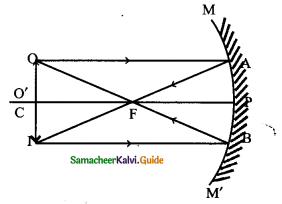(b) Position of an object: At C
Position of the image: At C

Nature of the image:
(i) Real
(ii) Inverted
(iii) Same size as the object

(ii) object At C and FPosition of the object: Between C and F
Position of the image: Beyond C

Nature of the image :
(i) Real
(ii) Inverted
(iii) Magnified

(iii) Object between F and P of the Mirror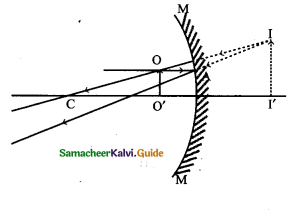Position of object : Between F and P
Position of the image : Behind the Mirror

Nature of the image :
(i) Virtual
(ii) Erect
(iii) MagnifiedQuestion 2.
Explain with diagrams how refraction of incident light takes place from a) rarer to denser medium b) denser to rarer medium c) normal to the surface separating the two media.
a) rarer to denser medium
When a ray of light travels from optically rarer medium to optically denser medium, it bends towards the normal.b) denser to rarer medium
When a ray of light from an optically denser medium to an optically rarer medium it bends away from the normal.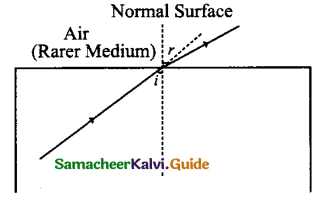c) normal to the surface separating the two media.
A ray of light incident normally on a denser medium it goes without any deviation.IX. Numerical Problems:

Question 1.
A concave mirror produces three times magnified real image of an object placed at 7 cm in front of it. Where is the image located?
Here given magnification m = 3
Object distance u = -7 cm
Magnification m = $$-\frac{v}{u}$$ Real image
-3 = $$-\frac{v}{u}$$
3u = -v
v = 3u = 3 x 7 = 21 cm
The image will be formed at a distance of 21 cm in front of concave mirror from its pole.

Question 2.
Light enters from air into a glass plate having a refractive index of 1.5. What is the speed of light in glass?
Refractive index of a glass plate μ = 1.5
Speed of light in vacuum is C = 3 x 108ms-1
Speed of light in glass V =?speed of light in glass = 2 × 108ms-1Question 3.
The speed of light in water is 2.25 × 108ms-1. If the speed of light in a vacuum is 3 × 108ms-1, calculate the refractive index of water.
Speed of light in water V = 2.25 × 108ms-1
Speed of light in vacuum C = 3 × 108ms-1
Refractive index of water μ =?X. Higher Order Thinking Skills.

Question 1.
Lightray emerges from water into the air. Draw a ray diagram indicating the change in its path in the water.Question 2.
When a ray of light passes from air into glass, is the angle of refraction greater than or less than the angle of incidence?
Light bends towards the normal because glass is denser than air.
It bends towards normal since light has to travel with the lesser speed in the glass but within a short time.
r < i. The angle of refraction is less than the angle of incidence.

Question 3.
What do you conclude about the speed of light in diamond, if the refractive index of diamond is 2.41?
Refractive index of diamond μ = 2.41∴ Speed of light in a diamond (1.24 × 108ms-1) is less than the speed of light in air (3 × 108ms-1).
The refractive index of diamond is 2.42, it means that speed of light in air (vacuum) is 2.42 times the speed of light in a diamond.

Intext Activities

ACTIVITY – 1

Stand before the mirror in your dressing table or the mirror fixed in a steel almirah. Do you see your whole body? To see your entire body in a mirror, the mirror should be atleast half of your height. Height of the mirror= Your height/2.
Solution :
(i) If the height of a person is 5 feet, then he should use a plane mirror of 2Vi feet height and fix in a steal almirah.
(ii) Now if he stand before it his full body will be seen on the mirror because hight of the mirror = $$\frac{\text { Our hieight }}{2}$$ACTIVITY – 2

Hold a concave mirror in your hand (or place it in a stand). Direct its reflecting surface towards the sun. Direct the light reflected by the mirror onto a sheet of paper held not very far from the mirror. Move the sheet of paper back and forth gradually until you find a bright, sharp spot of light on the paper. Position the mirror and the paper at the same location for few moments. What do you observe? Why does the paper catch fire?
Solution :
A concave mirror converges all the light rays coming from the Sun. All these light rays converge and meet at the focus of the mirror.
So, all the heat and light is focused on the principal focus (F). When a paper is kept at the focus (F), it starts burning, as this point is very hot.

ACTIVITY – 3

Take a convex mirror. Hold it in one hand. Hold a pencil close to the mirror in the upright position in the other hand. Observe the image of the pencil in the mirror. Is the image erect or inverted? Is it diminished or enlarged? Move the pencil slowly away from the mirror. Does the image become smaller or larger? What do you observe?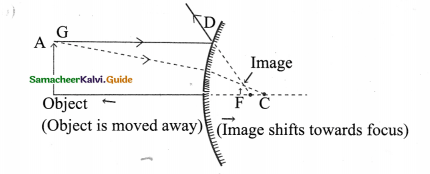Solution :

1. When a pencil is held in the upright position in front of a convex mirror, its diminished, erect image is formed which is virtual and therefore seen in the convex mirror.
2. When the pencil is moved away from the convex mirror size of image becomes smaller and smaller but image remains erect.
3. As we move away, the object from the convex mirror, image shifts towards the focus.ACTIVITY – 4

Refraction of light at air-water interface
Put a straight pencil into a tank of water or beaker of water at an angle of 45° and look at it from one side and above. How does the pencil look now?
The pencil appears to be bent at the surface of water.
Solution :Both the above activities are the result of refraction of light. The bending of light rays when they pass obliquely from one medium to another medium is called refraction of light.

I. Choose the correct answer :

Question 1.
A ray of light is incident towards a plane mirror at an angle of 30° with the mirror surface. What will be the angle of reflection?
(a) 45°
(b) 30°
(c) 90°
(d) 60°
(d) 60°Question 2.
A 10 mm long bin is placed vertically in front of a concave mirror. A 5 mm long image of the bin is formed at 30 cm in front of the mirror. The focal length of this mirror is
(a) -20cm
(b) -30cm
(c) -60cm
(d) -40cm
(a) – 20cm

Question 3.
A ray of light as it travels from medium A to medium B refractive index of the medium B relative to medium A is (μB/ μA)
(a) $$\frac{\sqrt{3}}{\sqrt{2}}$$
(b) $$\frac{\sqrt{2}}{\sqrt{3}}$$
(c) $$\frac{{1}}{\sqrt{2}}$$
(d) $$\sqrt{2}$$
(b) $$\frac{\sqrt{2}}{\sqrt{3}}$$Question 4.
Under which of the following conditions a concave mirror can form an image larger than the actual object?
(a) when the object is kept at a distance equal to its radius of curvature.
(b) when object is kept at a distance less than its focal length.
(c) when object is placed between the focus and centre of curvature
(d) when object is kept at a distance greater than its radius of curvature.
(c) when object is placed between the focus and centre of curvature

Question 5.
In torches, searchlights and head lights of vehicles the bulb is placed …………………………… of the concave mirror.
(a) between and F of the reflector
(b) Very near to F
(c) between F & C
(d) at C
(b) Very near to F

Question 6.
A boy is standing at a distance of 3m in front of a plane mirror. The distance between the boy and his image is ………………….. m
(a) 4
(b) 2
(c) 3
(d) 6
(c) 3Question 7.
The image formed by a concave mirror is real, inverted and of the same size as that of the object the position of the object should be
(a) beyond C
(b) between C & F
(c) at C
(d) at F
(c) at C

Question 8.
Which of the following has the highest refractive index
(a) air
(b) water
(c) diamond
(d) glass
(c) diamond

Question 9.
The image formed by a plane mirror is
(a) real
(b) diminished
(c) enlarged
(d) laterally inverted
(d) laterally invertedQuestion 10.
The incident ray passing through ‘F of a mirror ………………….. after reflection
(a) passes through C
(b) passes through F
(c) passes parallel to the principal axis
(d) passes through the pole
(c) passes parallel to the principal axis

Question 11.
The incident ray passing through C of a mirror ………………….. after reflection.
(a) passes through C
(b) passes through F
(c) passes through P
(d) parallel to the principal axis
(a) passes through C

Question 12.
The incident ray parallel to the principal axis of a mirror ………………….. after reflection.
(a) passes through C
(b) passes through F
(c) passes through P
(d) reverts back in the opposite direction
(b) passes through FQuestion 13.
According to sign convention the distance of the object.
(a) is always positive
(b) is always negative
(c) maybe positive or negative
(d) is equal to object height.
(b) is always negative

Question 14.
According to sign convention the distance of the image.
(a) is always positive
(b) is always negative
(c) maybe positive or negative
(d) is equal to image height
(c) maybe positive or negative

Question 15.
Total internal reflection will occur if the angle of reflection is
(a) 45°
(b) 60°
(c) 90°
(d) 99°
(d) 99°Question 16.
Magnification for the………………….. image is always …………………..
(a) real, positive
(b) real, negative
(c) virtual, negative
(d) virtual, positive
(b) real, negative

Question 17.
If magnification is +1.5. The image is …………………..
(a) erect
(b) diminished
(c) real
(d) invected
(a) erect

Question 18.
The refractive index of a denser medium with respect to rarer medium is
(a) 1
(b) greater than 1
(c) less than 1
(d) negative
(b) greater than 1Question 19.
We can see objects because of
(a) reflection
(b) refraction
(c) transmission
(d) diffraction
(a) reflection

Question 20.
The image formed by a convex mirror is always
(a) real (b) enlarged
(c) virtual & enlarged
(d) diminished
(d) diminishedQuestion 21.
As you move an object always from a convex mirror, its image becomes…………………..
and moves towards
(a) smaller, infinity
(b) smaller, focus
(c) enlarged, infinity
(d) enlarged, focus
(b) smaller, focus

Question 22.
For a spherical mirror ………………….. is true.
(a) f = 2R
(b) R = 2f
(c) fR = 2
(d) fR = $$\frac { 1 }{ 2 }$$
(b) R = 2fQuestion 23.
The mirror formula is…………………..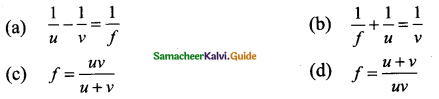(c) $$\frac{1}{f}=\frac{1}{u}+\frac{1}{v}$$

Question 24.
For a plane mirror, magnification m =
(a) 0
(b) 1
(c) ± 1
(d) ≤0
(b) 1

Question 25.
Magnification for convex mirror is
(a) always positive
(b) always negative
(c) some times positive
(d) 1
(a) always positive

Question 26.
If the angle of incidence i = 0, the angle of reflection r =
(a) 0°
(b) 90°
(c) 180°
(d) 45°
(a) 0°Question 27.
Refractive index of a medium is …………………..
(a) speed of light in air to speed of light in vacuum
(b) speed of light in vacuum to speed of light in air
(c) focal length to object distance
(d) speed of light in the medium × speed of light in the air
(a) speed of light in air to speed of light in vacuum

Question 28.
Bending of light as it passes from one medium to another is called
(a) reflection
(b) diffraction
(c) refraction
(d) deviation
(c) refraction

Question 29.
Ratio of sine of angle of incidence to sine of angle of refraction is ………………….
(a) gravitational law
(b) law of reflection
(c) law of refraction
(d) snell’s law.
(d) snell’s lawQuestion 30.
Entire light is reflected back into denser medium is called
(a) total internal reflection
(b) refraction
(c) reflection
(d) total external refraction.
(a) total internal reflection

Question 31.
Outer concentric shell in optic fiber is called
(b) core
(c) mantel
(d) coat

Question 32.
When light is going from a denser to a less dense medium, the critical angle is the angle of incidence for which the angle of refraction is
(a) 48°
(b) 90°
(c) 42°
(d) 51°
(b) 90°

Question 33.
A bundle of glass threads, each of which is capable of transmitting messages using light waves is called
(a) microscope
(b) convex
(c) periscope
(d) optic fibre
(d) optic fibreQuestion 34.
A ray of light travelling in medium 1 strikes and travels into another transparent medium 2. If the speed of light is greater in medium 1, the ray will
(a) refract towards the normal
(b) have an angle of incidence smaller than be angle of refraction
(c) refract away from the normal
(d) undergo total internal reflection
(a) refract towards the normal

Question 35.
A ray of light travels from air into a glass block as shown. It makes an angle of 30° with the surface of the block. If the refractive index of the glass is 1.5, what will be the angle of refraction?(a) 35.26°
(b) 1.30°
(c) 48.59°
(d) 19.47°
(d) 19.47°

Question 36.
The field of view is maximum for …………………
(* FOV is the extent of the observable area that is seen at any given instant)
(a) plane mirror
(b) concave mirror
(c) convex mirror
(c) convex mirrorQuestion 37.
A real and enlarged image can be obtained by using a
(a) convex mirror
(b) plane mirror
(c) concave mirror
(c) concave mirror

Question 38.
Which of the following statements about total internal reflection is true?
(a) angle of incidence should be greater than the critical angle
(b) light must travel from a medium of higher refractive index to a medium of lower refractive index
(c) both (a) and (b)
(c) both (a) and (b)

Question 39.
The focal length of a concave mirror is 5cm. Its radius of curvature is
(a) 5 cm
(b) 10 cm
(c) 2.5 cm
(b) 10 cmII. Fill in the blanks :

1. The ratio of the sine of the angle of incidence to the sine of …………………is constant.
angle of refraction

2. A spherical mirror whose reflecting surface is curved outwards is called ………………… mirror.
convex

3. All distances parallel to the principal axis are measured from the ………………… of the
mirror.
pole

4. A negative sign in the value of magnification indicates that the image is …………………
real

5. Light is refracted or bent while going from one medium to another because of its ………………… changes.
speedIII. State whether true or false. If false, correct the statement:

1. The critical angle is defined as the angle of incidence at which the total internal reflection starts to occur.
True.

2. The angle of incidence is equal to the angle of reflection for perfect reflection.
True.

3. The image formed in a plane mirror is always inverted.
False.
Correct statement: The image formed in a plane mirror is always erect.

4. A star appears twinkling into the sky because of the reflection of light by the atmosphere
False.
Correct statement: A star appears twinkling into the sky because of the refraction of light by the atmosphere.

5. Mirage is an example of refraction and total internal reflection of light.
True.6. Optical Fibres are based on the phenomenon of dispersion
False.
Correct statement: Optical Fibres are based on total internal reflection.

7. A water tank appears shallower when it is viewed from the top due to refraction.
True.

8. Twinkling of stars and Mirage are the two phenomena occurring due to refraction.
True.
Twinkling of stars and Mirage.

9. Angle of incidence is zero if a ray of light is incident normal to be surface separating the low media.
True.

10. A real image is inverted and can be caught on the screen.
True.

11. The minimum length of the mirror required to see the full image of the person is half ‘ of his height.
true.12. The pencil appears to be bent at the surface of the water is due to refraction.
True.

13. The speed of light decreases in a denser medium, light bends towards the normal.
True.

14. If the object is at infinity in front of a convex mirror the image is formed at infinity.
False.
Correct statement: The image is formed at F, behind the mirror

15. An object is placed at a distance of 3cm from a plane mirror. The distance of the object and image is 3cm.
False.
Correct statement: The distance of the object and image is 6 cm.

16. The distance from centre of curvature of the mirror to the pole is called the focal length of the mirror.
False.
Correct statement: The distance between the centre of the mirror and the focal point of the mirror is called the focal length of a mirror.17. Light is one of the slowest travelling energy with a speed of 3 × 10-8ms-1
False.
Correct statement : (Light is one of the fastest travelling energy with a speed of 3 × 10-8ms-1

18. The angle of incidence at which the angle of refraction is Q£ is called the critical angle.
False.
Correct statement: The angle of incidence at which the angle of refraction is 90° is called the critical angle.

IV. Match the following :

Question 1.

 Column I Column II (i) A plane mirror (a) Image is erect & smaller in size than the object. (ii) A concave mirror (b) Image is erect & of the same size as of the object. (iii) A convex (c) Used by dentists to see an enlarged images of teeth. (d) Can form images of objects spread over a large area.

 Column I Column II (i) A plane mirror (b) Image is erect & of the same size as of the object. (ii) A concave mirror (c) Used by dentists to see enlarged image of teeth. (iii) A convex (d) Can form image of objects spread over a large area.

Question 2.

 Column I Column II (i) r > 90 (a) Light gazes the surface of separation between two mode. (ii) r = 90 (b) No refraction. (iii) r < 90 (c) Refracted ray away from the normal

 Column I Column II (i) r > 90 (b) No refraction. (ii) r = 90 (a) Light gazes the surface of separation between two mode. (iii) r < 90 (c) Refracted ray away from the normalQuestion 3.

 Column I Column II (i) plane mirror (a) Focal length is positive. (ii) concave (b) Focal length is negative. (iii) convex (c) Focal length is infinity. (iv) Real image (d) Magnification if positive value. (v) Virtual image (e) Magnification if negative value.

 Column I Column II (i) plane mirror (c) Focal length is infinity. (ii) concave (b) Focal length is negative. (iii) convex (a) Focal length is positive. (iv) Real image (e) Magnification if negative value. (v) Virtual image (d) Magnification if positive value.

Question 4.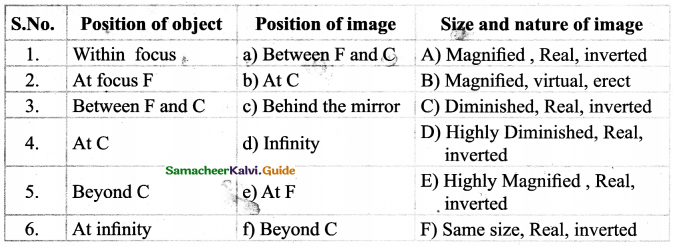V. Assertion & Reason Type :

(a) If both assertion & reason are true and the reason is the correct explanation of the assertion.
(b) If both assertion & reason are true but the reason is not correct explanation of the assertion.
(c) If assertion is true but reason is false.
(d) If assertion & reason both are false.
(e) If assertion is false but reason is true.Question 1.
Assertion : The air bubble shines in water.
Reason : Air bubble shines due to refraction of light.
(c) If assertion is true but reason is false

Question 2.
Assertion : The focal length of the mirror is /and distance of the object from the
focus is V then the magnification of the mirror will be $$\left(\frac{f}{f-u}\right)$$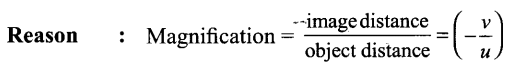(a) Both assertion & reason are true and the reason is the correct explanation of the assertion

Question 3.
Assertion : When an object is placed between two plane mirrors, then all the images found are of equal brightness.
Reason : Only two images are obtained in case of plane-parallel mirrors.
(d) Assertion & reason both are falseQuestion 4.
Assertion: The mirrors used in torch lights are parabolic not concave.
Reason: The image formed by concave mirror is always virtual.
(c) Assertion is true but reason is false

Question 5.
Assertion : The nature of the image depends on the size of the mirror.
Reason : Small mirrors always form a virtual image.
(d) Assertion & reason both are false

Question 6.
Assertion : A real image cannot be produced by plane or convex mirror.
Reason : The focal length of a convex mirror is always taken as possitive.
(e) Assertion is false but reason is trueQuestion 1.
Which is optically denser out of the two medium M1& M2 having the refractive indices = 1.71 and 1.36 respectively?
M1
Reason: Optical density increases as the value of the refractive index increases.

Question 2.
Two medium with refractive index 1.31 & 1.50 is given. In which case (i) Bending of light is more and (ii) speed of light is more.
(i) 1.50 – Bending is more
(ii) 1.31 – Speed is moreQuestion 3.
Under what circumstances there won’t be any refraction of light when it enters from one medium to another?

• When light incident at 90°, it will not bend.
• When light passes from denser medium to rarer medium and if it is incident at an angle greater than the critical angle, it will reflect but will not refract.

Question 4.
A ray of light traveling in air enters obliquely into water. Does the light ray bend towards the normal or away from the normal? Why?

• When a ray of light travels from air into water obliquely, it bends towards the normal.
• This is because water is optically denser than air.
• On entering water, speed of light decreases and the light bends towards normal.

Question 5.
List down the uses of concave mirror.
Concave mirrors are used :

• As Shaving mirror.
• As Reflectors in automobile headlights & torches.
• By dentists to see large images of the teeth of patients.
• To produce heat in solar furnaces.Question 6.
What are the characteristics of the image formed on a plane mirror?

• Image is upright.
• Size of the image is the same as the size of the object.

Question 7.
State the laws of reflection of light.

• The incident ray, the normal at the point of incidence and the reflected ray, all lie in the same plane.
• Angle of incidence is equal to the angle of reflection.

Question 8.
Describe the nature of images formed by plane mirrors.

• It is always virtual and erect.
• Its size is equal to that of the object
• It is formed at the same distance behind the mirror as the object is in front of the mirror.
• It is laterally inverted.Question 9.
What is lateral inversion in a plane mirror?
It means the apparent reversal of left and right in the mirror image compared with the object.

Question 10.
Explain why a ray of light passing through the centre of curvature of a concave mirror, gets reflected along with the same pattern.
The ray passing through the centre of curvature incident to the mirror along its normal so < i = < r = 0
∴ The ray retraces its pattern

Question 11.
How tall does a mirror have to be to fit an entire person’s body?
The height of the mirror should behalf of the person’s height regardless of the distance of the person from the mirror.

Question 12.
What is concave and convex mirror?

• If the reflecting surface is curved inwards that is called concave mirror.
• The reflecting surface is curved outwards, then it is called convex mirror.Question 13.
Define principal focus of concave mirror.
Principal focus (F): The point on the principal axis of the spherical mirror where the rays of light parallel to the principal axis meet or appear to meet after reflection from the spherical mirror.

Question 14.
What is focal length (f) of a mirror?
The distance between the pole (P) and the principal focus (F) of the spherical mirror is called the focal length.
f = $$\frac { R }{ 2 }$$ , where R is the radius of curvature of the mirror.

Question 15.
The radius of the hollow sphere of which the spherical mirror forms a part is called the radius of curvature (R)
R = 2 × focal lengthQuestion 16.
What is “aperture”?
The diameter of the circular rim of the mirror is called the aperture of the mirror. It is the entire area of the reflecting surface of the mirror.

Question 17.
Distinguish between real & virtual image.
Real :

1. Light rays that come from an object actually meet after reflection.
2. It can be obtained on a screen
3. Image is inverted

Virtual :

1. Light comes from an object does not actually meet but appears to meet.
2. It cannot be obtained on the screen.
3. The image is erect.Question 18.
What do you mean by linear magnification?
It is defined as the ratio of the height of the image (hi) to the height of the object (ho).
$$m=\frac{h_{i}}{h_{o}}(\text { or }) \quad m=-\frac{v}{u}$$

Question 19.
Which kind of mirrors are used in the shaving mirror? Why?
Cancave mirror, to get magnified image. When a cancave mirror is held near the face (between the pole and focus of the mirror) then an upright and magnified image is seen.

Question 20.
Which mirror is used as a reflector? Why?

• Concave mirrors are used as reflectors in torches, vehicle headlights and searchlights.
• To get a powerful parallel beam of light.

Question 21.
Write the uses of cancave mirror

1. As a shaving mirror: Magnified face will be seen
2. As a dentist head mirror: Focuses the lights on to see a small area of the body (teeth, throat, etc.)
3. As reflectors: To get a powerful beam of light
4. In solar heaters: To focus sun light for heaters

Question 22.
What do you observe when an object is placed anywhere between P and infinity in front of a convex mirror?

• The image is formed behind the mirror between the pole (P) and focus (F).
• Virtual and erect image.
• Diminished image.Question 23.
What is the nature of the image formed by a concave mirror if the magnification produced by the mirror is +4?
Positive sign of magnification indicates that image is virtual, erect and enlarged.

Question 24.
Between which two points of a concave mirror should an object be placed to obtain a magnification of -2?
Negative sign of magnification indicates that image is real and inverted. Sign of image is magnified (enlarge)
∴ Object is placed between F and C.

Question 25.
To obtain an image twice the size of the object, between which two points related to a concave mirror should an object be placed?
Between F (principal focus) and C centre of curvature.
Real Image.

Question 26.
Draw a ray diagram and also state the position the relative size and nature of image formed by a concave mirror, when an object is placed at C.
Image Position: At C, itself
Size: Same size
Nature : (i) Real
(ii) Inverted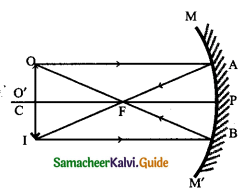Question 27.
Why a pencil partly immersed in water appears to be bent at the water surface.
The pencil dipped in water appears bent due to the refraction of light.
The angle of refraction depends on the refractive index of the medium.

Question 28.
How should a ray of light be incident on a rectangular glass slab so that it comes out from the opposite side of the slab without being displaced?
Along the normal to the surface or at an angle of incidence of i = 0.

Question 29.
Why a convex mirror is preferred for rearview mirrors in cars?

• It always forms virtual, erect, and diminished images.
• The field of view increases.

Question 30.
List four properties of the image formed by a convex mirror.

• Always formed behind the mirror between P & F.
• Virtual & erect
• Size is always smaller than the object.
• Magnification is always positive.Question 31.
List four properties of the image formed by the concave mirror when the object is placed between F & P

• The image formed behind the mirror
• Enlarged
• Virtual
• Erect

Question 32.
What is meant by the refraction of light?
Light does not travel in the same direction in all media. It changes its direction, the bending of a light ray when it passes from one medium to another is called refraction of light.

Question 33.
State the laws of refraction of light.
(i) The incident ray, the refracted ray, and the normal to the interface of two transparent media at the point of incidence, all lie in the same plane.

(ii) The ratio of the sine of the angle of incidence to the sine of the angle of refraction is a constant for a light of a given colour and for the given pair of media. This law is also known as Snell’s law of refraction.
If i is the angle of incidence and r is the angle of refraction, then $$\frac{\sin i}{\sin r}[latex] = constant This constant is called the refractive index of the second medium with respect to the first medium. TQuestion 34. Define refractive index & write its unit. Answer: The refractive index of the second medium with respect to the first medium is defined as the ratio of the sine of the angle of incidence in the first medium to the sine of the angle of refraction in the second medium. Unit: The refractive index has no unit as it is the ratio of two similar quantities Question 35. Define refractive index in terms of speed of light. Answer: The refractive index of a medium is also defined in terms of speed of light in different media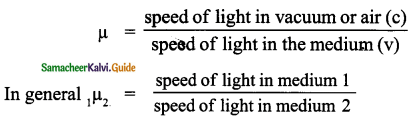Question 36. What is total internal reflection? Answer: Total internal reflection: When the angle of incidence exceeds the value of the critical angle, the refracted ray is not possible, since r > 90°, the ray is totally reflected back to the same medium (denser medium). This is called as total internal reflection.Question 37. Define the critical angle. Answer: The angle of incidence at which the angle of refraction is 90° is called the critical angle. Question 38. What are the conditions to achieve total internal reflection? Answer: • Light must travel from denser medium (Water) to rarer medium (Air). • The angle of incidence inside the denser medium must be greater than that of the critical angle. Question 39. What is mirage? How it occurs? Answer: • Mirage is an optical illusion caused by the refraction of light from the sky by heated air. • The air near the ground becomes hotter than the air at higher levels. • The refractive index of air increases with its density. Hotter air is less dense, and has smaller refractive index than cooler air. • If the air currents are small, that is, the air is still, the optical density of different layers of air increases with height. • As a result, light from an object such as a car passes through a medium whose refractive index decreases towards the ground. • Thus, a ray of light from such an object successively bends away from the normal and undergoes total internal reflection, if the angle of incidence for the air near the ground exceeds the critical angle.Question 40. How do twinkling stars occur? (or) what is the cause of the twinkling of stars? Answer: Refraction of light: • Light from a star is refracted as it passes through our atmosphere because the light passes rapidly through moving cells of air with different densities, temperatures, etc. • These cells bend light by different amounts and make the apparent position of the star move around. This movement is seen as twinkling. Question 41. What is the phenomenon used in optical fibre? Explain. Answer: • Optical fibres are extensively used for transmitting audio and video signals through long distances. • Optical fibres too make use of the phenomenon of total internal reflection. • Optical fibers are fabricated with high-quality composite glass/quartz fibres. Each fibres consists of a core and cladding. The refractive index of the material of the core is higher than that of the cladding. When a signal in the form of light is directed at one end of the fibre at a suitable angle, it undergoes repeated total internal reflection along the length of the fibre and finally comes out at the other end. Optical fibres are extensively used for transmitting and receiving electrical signals which are converted to light between the ends of the fibre, over long distances.Question 42. Write any two uses of total internal reflection. Answer: 1. Spectacular brilliance of diamonds. 2. Transmission of audio and video signals to long-distance through optical fibres. Question 43. What are the examples of total internal reflection in nature? Answer: 1. Mirage 2. Twinkling of stars Question 44. Give two examples of the transparent medium that are denser than air. Answer: Water, glass. Question 45. A coin in a glass beaker appears to rise as the beaker is slowly filled with water why? Answer: Refraction of light at the air-water interface.Question 46. Name the spherical mirror(s) that has/have (i) Virtual principal focus (ii) Real principal focus Answer: (i) Convex (ii) Concave. VII. Long Answers Question 1. List the sign conventions for reflection of light by spherical mirrors. (i) Write the formula for the spherical mirror. (ii) Mirror Equation Answer: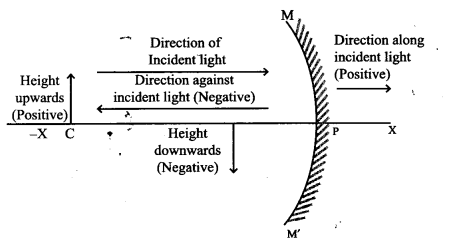(i) • The object is always placed on the left side of the mirror. • All distances are measured from the pole of the mirror. • Distances measured in the direction of light are taken as positive (along + X-axis) and those measured in the opposite direction are taken as negative (along – X-axis). • All distances measured perpendicular to and above the principal axis (along + Y-axis) are considered to be positive. • All distances measured perpendicular to and below the principal axis (along – Y-axis) are considered to be negative. Cartesian sign conventions are used to derive mirror formula and do simple calculations.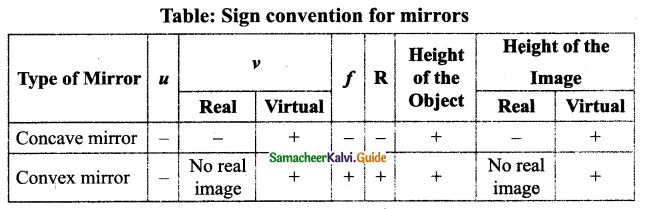(ii) Mirror Equation The expression relating the distance of the object u, a distance of image v, and focal length f of a spherical mirror is called the mirror equation. [latex]\frac{1}{f}=\frac{1}{u}+\frac{1}{v}$$

f – focal length of a spherical mirror
u – Distance of the object
v – Distance of the image

Linear Magnification:

It can be defined as the ratio of the height of the image (hi) to the height of the object (ho)
m = $$\frac{h_{i}}{h_{o}}(\text { or }) \frac{-v}{u}$$
hi = height of the image
ho = height of the object

Question 2.
State the type of mirror used as
(i) Convex mirror
(ii) Concave mirror
(i) Convex mirror :

• It gives a wide field of view
• It produces erect and small size image of the object. [As the vehicles approach the driver from behind, the size of the image increases. When vehicles are moving away from the driver, the image size decreases]

(ii) Concave mirror:
To see a large size image of the face.
When the object lies in between pole and principal focus, it forms a virtual, erect and enlarged image.Question 3.
Write the rules for the construction of image by Concave mirrors, along with ray diagram. .
(i) Rule 1 : A ray passing through the centre of curvature is reflected back along its own path.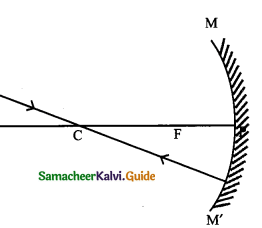(ii) Rule 2 : A ray parallel to the principal axis passes through the focus after reflection.(iii) Rule 3 :A ray passing through the focus gets reflected and travels parallel to the principal axis.(iv) Rule 4 : A ray AP incident at the pole of the mirror gets reflected along a path PB
such that the angle of incidence APC is equal to the angle of reflection BPC.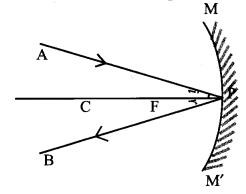Question 4.
Describe the nature and location of the images for the different positions of object which is placed in front of the concave mirror.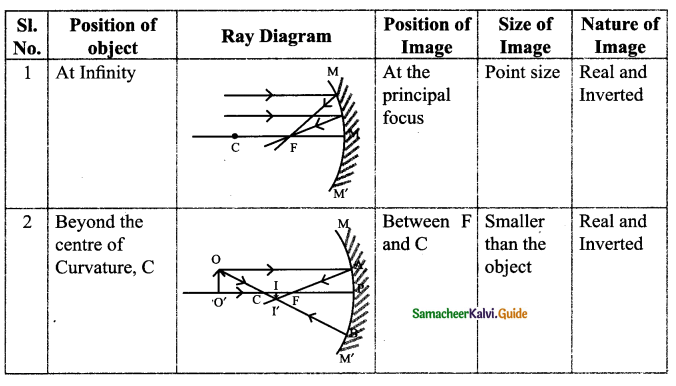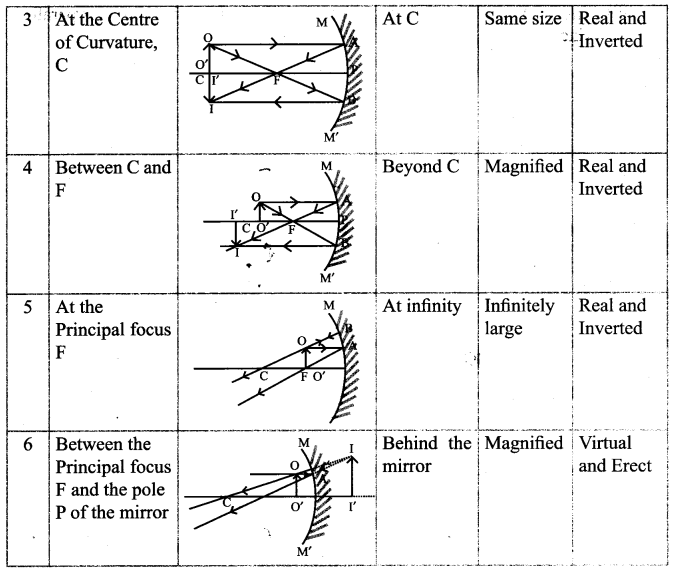VIII. Higher-Order Thinking Skills :

Question 1.
M = 2.42 for diamond. What is the meaning of this statement in relation to the speed of light?